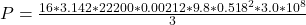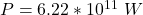## Someone plans to float a small, totally absorbing sphere 0.518 m above an isotropic point source of light, so that the upward radiation forc

Question

Someone plans to float a small, totally absorbing sphere 0.518 m above an isotropic point source of light, so that the upward radiation force from the light matches the downward gravitational force on the sphere. The sphere’s density is 22.2 g/cm3, and its radius is 2.12 mm. (a) What power would be required of the light source

in progress 0
3 months 2021-07-29T14:11:56+00:00 1 Answers 2 views 0

Explanation:

From the question we are told that

The  height is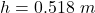The  sphere density is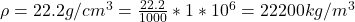The  radius is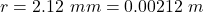Generally the power required  is mathematically represented as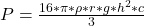substituting values